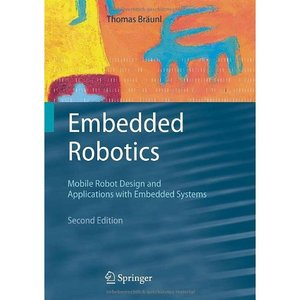Wednesday, September 12, 2012

Advanced Embeded Robotics PPT and PDF SLIDESAdvanced Embeded Robotics
Instructor: Marke A.Perkowski
Textbook: Embedded Robotics , Thomas Braunl

PROPOSITIONAL LOGIC AND MODAL LOGIC IN ROBOTICS.

KNOWLEDGE REPRESENTATION AND PLANNING WITH FIRST ORDER LOGIC.

Mobile robots.

PROBABILISTIC ROBOTICS. LOCALIZATION. PLANNING. MAPPING.

Robot Path Planning and related topics.

===============================================================

STATIONARY ROBOTS.

1. Motion Planning for stationary robots.

ROBOT SOCCER AND SIMILAR IMPROVISATIONS.

Matrix Calculations

ROBOT COMPETITIONS, ROBOT TEAMS, AND ROBOT SOCIETIES.

VARIOUS ROBOTIC ARCHITECTURES.

Emotional Robots.

1. Slides in PPT by Mark Brosnan about Affective computing. This material related to class project about robot theatre.

WALKING ROBOTS.

Gaits for Walking robots

HUMAN-ROBOT AND HUMAN-COMPUTER INTERACTION.

Cameras and Visual Servoing

BASIC ROBOT VISION.

Tracking.

MATLAB AND MATLAB IN ROBOT VISION.

1. matlab1.pdf Lectures on Matlab. Lecture 1. Introduction to Matlab.

2. matlab2.pdf Lectures on Matlab. Lecture 2. More Matlab Programming.

3. matlab3.pdf Lectures on Matlab. Lecture 3. Finishing with Matlab.

4. matlab4.pdf Lectures on Matlab. Lecture 4. Finishing with Matlab.

5. Lecture on Introduction and Control Basic to Matlab. In PDF.

6. The same lecture in PPT.

7. Matlab Primer in PDF.

8. Introduction to Matlab in PPT.

9. Matlab two-dimensional plots in PPT.

10. Matlab Script and Function files in PPT.

11. Simple Programming in Matlab in PPT.

12. Solution of non-linear algebraic equations in Matlab. PPT format.